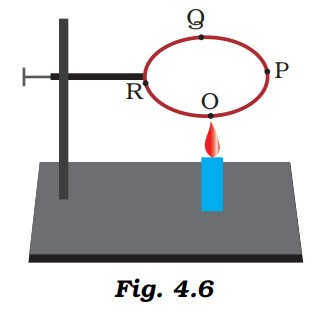#### A circular metal loop is heated at point O as shown in Figure 4.6.(i) In which direction would heat flow in the loop?(ii) In which order the pins at points P,Q and R fixed with the help of wax fall if points O, P, Q and R are equidistant from each other?1. The heat will flow in both the directions i.e. from O to P and O to R.

2. At first, the pins at R and P will fall simultaneously followed by the pin at Q.